5.7. Cooling flows and accretion by cDs

If the rate of cooling in the intracluster gas is sufficiently rapid, gas may cool and flow into the center of the cluster. Lea et al. (1973), Silk (1976), Cowie and Binney (1977), and Fabian and Nulsen (1977) noted that the cooling times tcool (equation 5.23) in the more luminous X-ray clusters are comparable to the Hubble time th (the age of the universe and approximate age of clusters). This might be a coincidence, but it would also be a natural consequence of the cooling of the intracluster gas. Imagine that a certain amount of gas was introduced into the cluster when it formed. Initially, the cooling time in this gas might be much longer than the age of the cluster, and cooling would be unimportant. However, as long as cooling was more rapid than heating, and the gas was not removed from the cluster, the cooling time would not increase, and eventually the age of the cluster would exceed the cooling time. Once this occurs, the gas will cool in the cluster core, and the pressure of the surrounding gas will cause the cool gas to flow into the cluster center. The surrounding hot intracluster gas will always have tcool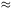th. Silk (1976) and Cowie and Binney (1977) showed that this cooling condition could explain a number of the observed correlations between the optical and X-ray properties of clusters, particularly the Lx -r relation (equation 4.8). The subject of these cooling flows has been reviewed recently by Fabian et al. (1984b).

Silk (1976) suggested that the intracluster gas was introduced into the cluster when it formed, and that cooling has reduced the amount of intracluster gas so as to maintain tcth. Cowie and Binney (1977), on the other hand, argued that the intracluster gas is constantly being replenished by ejection from cluster galaxies. Then, the density of gas in the cluster center would increase until the age exceeded the cooling time. After this point, a stable steady-state would be achieved, in which the rate of ejection of gas by galaxies into the cluster was balanced by the rate of removal of gas through cooling in the cluster core (Cowie and Binney, 1977; Cowie and Perrenod, 1978). As long as the mass ejection rate is not too low, cooling would regulate the inflow, and the densest hot gas at the cluster center would always have tcoolth.

The equations for a steady-state cooling flow in a spherically symmetric cluster are identical to those for a wind (equation 5.90), except that the heating rate h(r) is replaced by the cooling rate(equations 5.21 and 5.22). Because the cooling rate is proportional to the square of the density, it is useful to defineg2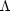(Tg). Then,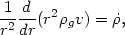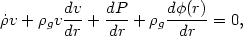(5.101)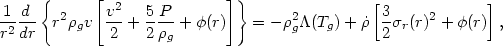and the other symbols are defined following equation (5.90). If the gas is injected continuously into the cluster (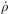= 0), then the boundary conditions are set by requiring no inflow from outside the cluster. If the gas is not constantly being added to the cluster (= 0), then no cluster-wide steady-state flow will be possible. However, these equations will still apply approximately within the radius defined by setting the cooling time equal to the age of the cluster (the 'cooling surface') . In order to match to the hydrostatic intracluster gas distribution beyond this radius, the proper density and temperature is specified on this surface. Because the inflow is generally extremely subsonic except in the innermost parts, the outer parts of these cooling flows are very nearly hydrostatic (the middle equation in 5.101 reduces to 5.55). The velocity is determined by the inflow rate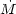4g vr2, which must be constant in a steady-state inflow without sources or sinks for mass.

Thermal conduction (Section 5.4.2) has not been included in the cooling flow equations. Generally, the existence of cooling flows requires that conduction not be very important, since otherwise cooling in the cluster core will be balanced by heat transported inwards by conduction, rather than heat convected inwards by the cooling flow (Mathews and Bregman, 1978; Takahara and Takahara, 1979; Binney and Cowie, 1981; Nulsen et al., 1982). In most cases, conduction must be much slower than the Spitzer rate (equation 5.37) for a nonmagnetic plasma; the suppression of conduction might be due to a very tangled or circumferential magnetic field (Section 5.4.3).

These equations have been solved for cooling in clusters by Cowie and Binney (1977), assuming a King model for the cluster density (equation 2.13), mass ejection by galaxies as given by Yahil and Ostriker (1973; equation 5.91), pure bremsstrahlung cooling (equation 5.21), and subsonic flow (dropping the quadratic velocity terms in equations 5.101). Figure 37 shows the density and temperature variations in a typical model. The density rises continuously into the center; this contrasts with hydrostatic models, in which it levels off (Section 5.5). The temperature has a maximum at about two core radii and declines into the cluster center. The behavior of these solutions in the inner regions can be understood approximately. Assuming that the mass flux is fixed (= const), that the flow is subsonic, that the gravitational potential is not important (so that the pressure is nearly constant and the flow is driven by pressure and cooling and not by gravity), and that the cooling function is a power-law in the temperatureTg, the temperature and density are found to vary asg1 / Tgr-3/(3-) (Nulsen et al., 1982). For Tg4 × 107, cooling is due to thermal bremsstrahlung (equation 5.21),= 1/2, andgr-6/5. At lower temperatures,-0.6 (equation 5.22) andgr-5/6. When the gas has cooled sufficiently for the gravitational potential to be important (or if the potential gradient is increased by a central galaxy), then the temperature tends to vary as kTg / µmpGM(r) / r, where m(r) is the total mass within r (Fabian and Nulsen, 1977).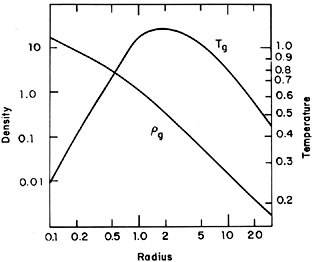Figure 37. The gas temperature and density in a cluster with a steady-state cooling flow, from the models in Cowie and Binney (1977). The gas density is normalized to its value at the cluster core radius, and the gas temperature is normalized to µmpr2 / k, wherer is the cluster velocity dispersion and µ is the mean atomic mass. The radius from the cluster center is in units of the cluster core radius.

Evidence for the existence of such cooling flows in X-ray clusters includes the detection of peaks in the soft X-ray surface brightness at the cluster center (Branduardi-Raymont et al., 1981; Fabian et al., 1981a; Canizares et al., 1983; Fabricant and Gorenstein, 1983; Jones and Forman, 1984; Stewart et al., 1984a, b), the measurement of inverted temperature gradients dTg / dr > 0 (Gorenstein et al., 1977; Ulmer and Jernigan, 1978; White and Silk, 1980), and the observation of central X-ray surface brightnesses and temperatures that imply cooling times much less than the Hubble time (Canizares et al., 1983; Stewart et al., 1984b). The strongest evidence comes from the detection of soft X-ray line emission from low ionization stages produced at temperatures of Tg106 - 107 K, coming from the cluster center (Canizares et al., 1979, 1982; Canizares, 1981; Mushotzky et al., 1981; Lea et al., 1982; Nulsen et al., 1982; Culver et al., 1983; Mushotzky, 1984; Section 4.3.3).

Theoretical models for cooling flows can be used to estimate the rates of cooling in clusters from these X-ray observations. A simple estimate may also be derived by noting that for steady-state, isobaric cooling, the luminosity emitted in any temperature range dTg is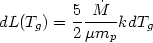(5.102)

if the gravitational potential does not change significantly during the cooling (Cowie, 1981). Here, µ is the mean atomic mass. This equation can be used to estimate the luminosity in any spectral feature produced in the cooling flow by integrating dL(Tg) over the fraction of the total emission in that feature as a function of temperature. A more accurate semi-empirical method of determining cooling rates for clusters has been derived Canizares et al. (1983), Stewart et al. (1984b), and Fabian et al. (1984b). This method is essentially that of Section 5.5.4. First, the X-ray surface brightness is deconvolved to give the X-ray emissivity as a function of position (equation 5.81). Second, the hydrostatic equation is used to derive the gas temperature as a function of position, assuming some form for the gravitational potential (equation 5.85). (Note that the hydrostatic equation holds for the outer portions of these cooling flows because they are very subsonic; see Section 5.7.2.) Third, the cooling time is calculated in the gas, and the gas is assumed to form a steady-state cooling flow within the cooling surface at which tcoolth. Finally, the steady-state energy equation (the last of equations 5.101) is used to determine the cooling rate.

Table 4 gives a list of clusters showing evidence for cooling flows, with estimates for the cooling rate. The values forrange from 3 - 1000 M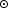/ yr. The Perseus cluster has one of the largest observed cooling rates (see also Section 4.5.2). A very small cooling rate of 3 - 20 M/ yr is found in M87 in the Virgo cluster; such a small cooling flow would be difficult to observe in more distant clusters. It is particularly interesting that cooling flows have now been detected in poor clusters (Canizares et al., 1983), as well as in a large number of rich clusters (Stewart et al., 1984b).

It should perhaps be pointed out that all of the above evidence shows that there is cool gas in the cores of these clusters, but it does not directly show that there is cooling gas flowing into the cluster core. That is, the motion of the gas has not been detected directly, as X-ray spectral observations have insufficient resolution to detect Doppler shifts produced by the slow inflow. It has occasionally been argued that the gas might actually be in hydrostatic and thermal equilibrium, with the extra emission at the cluster center being due to some central heat source (see, for example, Tucker and Rosner, 1983). Since the cooling rate of a gas at constant pressure decreases with temperature at all temperatures of interest for X-ray emission (105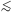Tg109 K), the higher the heating rate (or the nearer the cluster center), the cooler the gas would be in thermal equilibrium. However, such hydrostatic, thermal equilibrium models (in which one heats the gas in order to cool it) can generally be shown to be thermally unstable on the cooling time scale (Stewart et al., 1984a; Fabian et al., 1984b). The gas must either heat up and expand out of the core, or cool down and form a cooling flow on this time scale. Moreover, such a thermal equilibrium model for the X-ray emission would not explain the observed association of cooling flows with optical line emitting filaments, as discussed below.